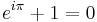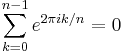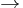# User:Jian

I am currently a PhD student at Prof. You's lab. During the winter of 2015, I am the TA of ChE 352. I am responsible for introducing the use of this websites, and grading the webpages contributed by the students. If you have any concerns, you are more than welcome to contact me any time you want.

Editing examples

what is going on here?

a bunch of disordered examples

----$e^{i \pi} + 1 = 0$$\sum_{k=0}^{n-1} e^{2 \pi i k/n} = 0$$\rightarrow$

Table 1. Example